# FDR and q-values

## illustrating John Storey's method

### by Alejandro Ochoa García

Computing the false discovery rate is easier than you imagine!

VIIIA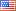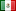-

## Introduction

John Storey created a method for turning a list of p-values into q-values, the difference being that a p-value measures the cumulative probability that a single test was assigned by a null model, while a q-value measures the False Discovery Rate (FDR) you would incur by accepting the given test and every test with a smaller p-value (and maybe even larger p-values, if they improve the FDR).

The main problem q-values set out to solve is the multiple hypothesis testing problem. The problem is that a p-value, long the standard of hypothesis testing, is not reliable when multiple tests are performed at the same time. This is a common problem in biology, where you might ask whether gene expression changes are significant over an entire genome (over 6,000 tests in yeast, or 20,000 in humans, corresponding to the number of genes). So in a single test you might reject the null hypothesis if the p-value is smaller than 0.05 (meaning the null hypothesis generates the observed or more extreme data with 5% chance), but with multiple tests, the chance that any of them will be false increases dramatically with the number of tests, so setting a p-value threshold of 0.05 leads to many more than 5% of the tests being false.

Let's assume what we really want is the value of the FDR, defined as the fraction of tests that pass a threshold but are false. At first approximation, it seems that correcting the p-values by multiplying them by the number of tests made should better approximate the desired FDR, and indeed this is what the Bonferroni correction does. Unfortunately this is overkill, in that it also makes you throw away too many good tests. More advanced corrections have been developed since (too many to list, I don't aim to be comprehensive here), but currently Storey's method seems to best balance the reduction of false tests with the increase of true tests.

Storey has produced many great theorems, and this page doesn't aim to replace them. Instead, I thought I could graphically complement the theory so that anybody interested in implementing the q-value procedure could intuitively understand what they're doing. I'm certainly ignoring subtelties in formulation, notation, etc; if you want the full math see (Storey, 2002). I prefer thinking in pictures.

## P-values of null tests have a uniform distribution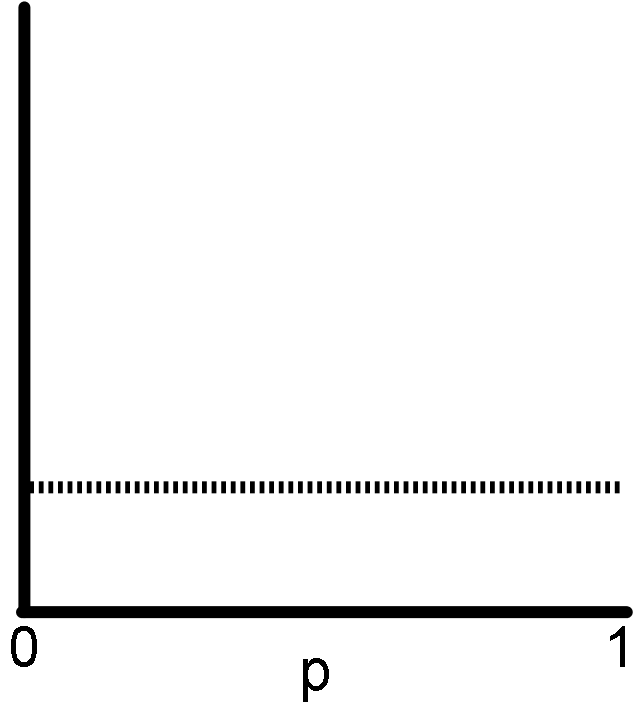This is only so hard to explain because it's supposed to be obvious. Imagine making multiple tests, every one of which you are certain (by construction?) the null hypothesis is true for. Make a histogram of the list of p-values you get. What proportion of them have p smaller than t? It should be t!

With real data you'd expect small fluctuations (not shown in my illustration), due to the stochastic nature of the data, but the trend should be a uniform distribution.

It is recommended that you run this test if you can. Gather a set where you expect the null hypothesis to be true, and if you don't see a uniform distribution, you can be sure you're not calculating your p-values correctly, or the null hypothesis you chose is incorrect. It's crucial to the procedure that p-values are correct!

## P-values of real tests should have a peak on zero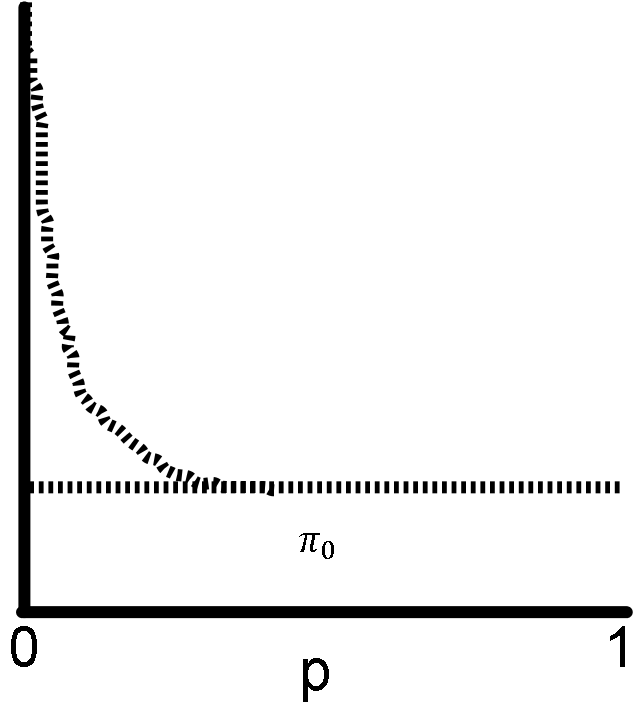The assumption here is that among your tests, the ones with the smallest p-values are enriched for tests in which the null hypothesis is false.

It is important that the distribution remains uniform away from p=0 (with small fluctuations, again not depicted), where the majority of the tests should conform to the null hypothesis. Otherwise the same caveats as before apply: either your p-values are not being computed correctly, or your null hypothesis is incorrect.

The key in Storey's procedure lies in estimating how many false predictions are near p=0. Imagine your p-value distribution is a mixture of two underlying distributions, one where the null hypothesis is true (uniform), and the other where the null hypothesis is false (the peak on zero).

The procedure needs the value of π0, the proportion of all tests in which the null hypothesis is true. In my figure, you need to find the height of the line that approximates the distribution of the null p-values. It is easiest to estimate this value by walking from p=1 towards p=0. The farther you are from p=1, the more data you are using in estimating π0, so the variance of the estimate is lower, but you risk including tests where the null hypothesis is false (therefore getting a value larger than the true π0).

If you feel you cannot reliably estimate π0, you can set it to 1. This will reduce the power of the FDR procedure, in that the real FDR will be smaller than your estimate, so you'd be losing true predictions. But usually π0 is very close to 1 (if most of the tests satisfy the null hypothesis). In fact, setting it to 1 reduces this part of the Storey procedure into the Benjamini and Hochberg procedure, the predecessor, and you obviously get the same answer both ways if π0 is close enough to 1.

## Getting the FDR from a p-value threshold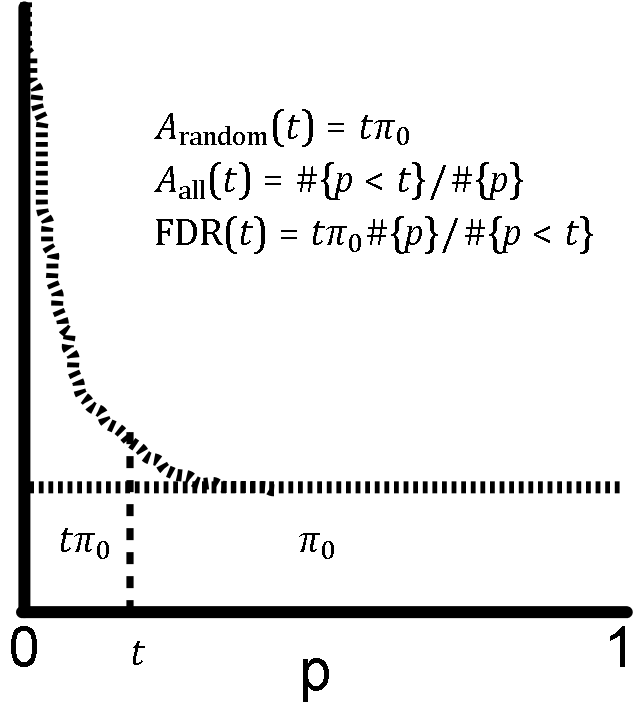Here we finally get the FDR from the problem of setting a threshold on the p-values.

Let t be the threshold on your p-values (each test with p < t will pass). The FDR has two parts. Assume the total area has been normalized to 1. The denominator is the total area with p < t, or the ratio of number of tests with p < t to the total number of tests. The numerator is the estimated area of false tests with p < t. To reiterate our previous findings, the total area of false tests is π0, and the fraction of that area with p < t, since it is uniform, is t⋅π0. The final formula, again the fraction of predictions that is false, is shown in the figure.

## Mapping p to q-values

So things are good if you've chosen t and want its FDR, but generally the FDR is chosen first, and we wish to find the threshold t that has such an FDR. Even better, can we analyze the data without even settling on a given FDR? Storey provides theorems that show we can do the following without any funny business.

First we produce the map of t to FDR(t). Essentially we walk from t=0 to t=1, and we store the FDR values as we go. This can be done quite efficiently if you code smartly. We could treat FDR(p) as the q-value of p, but we can do a bit better. Usually FDR(p) increases as you increase p (you can see that in the figures above), but this isn't always the case (imagine what happens if the real data fluctuates a lot). In that case, the FDR(t) will be smaller for a threshold t larger than the p we're looking at! It makes sense to use that FDR(t) as the q-value of p, since we will get more predictions and a lower FDR at the same time!

So q(p) = mint; p<t FDR(t) is the final definition of the q-value of p. This way, q-values are monotonic with p. To compute, once the t to FDR(t) map has been computed, we can walk t backwards, from 1 to 0, to apply this "minimum". Now, if you want an FDR of 0.05, you accept all predictions with q < 0.05. If you instead want an FDR of 0.01, you use q < 0.01. It's that easy!